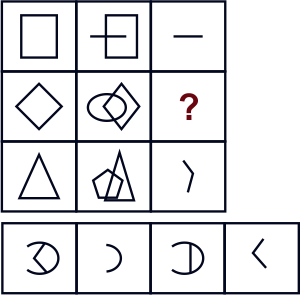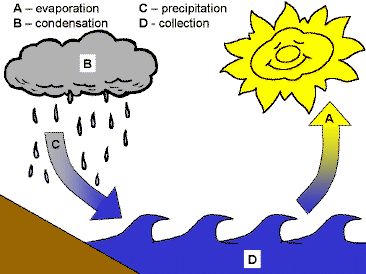# Humming Bird - HSO PDF Sample Papers for Class 9

Resources:

Class 9 sample paper & practice questions for Humming Bird Science Olympiad (HSO) level 1 are given below. Syllabus for level 1 is also mentioned for these exams. You can refer these sample paper & quiz for preparing for the exam.Q.1 Q.2 Q.3 Q.4 Q.5 Q.6 Q.7 Q.8 Q.9 Q.10
 Q.1 Ajit walks a distance of 3 km towards South, then turns to his left and walks 4 km. He again turns left and walks for 6 km. At this point he turns to his left and walks for 8 km and finally moves 3 km towards South. How many kilometres is he from the starting point? a) 4 b) 5 c) 6 d) 8
 Q.2 Where are the pores called 'Stomata' located in plants? a) Bark b) Roots c) Leaves d) Stems
 Q.3 What is the C.G.S unit of velocity? a) m/s b) cm/s c) cm/minute d) m/minute
 Q.4 Select a suitable figure from the four alternatives that would complete the figure matrix.a) 1 b) 2 c) 3 d) 4
 Q.5 Which of the following is the fundamental unit of human body? a) Cell b) Element c) Atom d) Ions
 Q.6 If R stands for addition, S stands for subtraction, T stands for multiplication, U stands for division, V stands for equal to, W stands for greater than, and X stands for less than, choose the correct answer. a) 15 U 5 R 3 V 2 T 3 b) 15 S 5 T 3 W 2 R 3 c) 15 U 5 W 3 R 2 T 3 d) 15 R 5 U 3 V 2 R 3
 Q.7 Law of constant proportion is also known as _______________. a) Law of definite proportions b) Proust’s Law c) Both a and b d) None of the above
 Q.8 The rectangular components of a force are applied to move a body. One of the components is 30 N. What should be the other component to obtain a resultant force of 50 N? a) 30 N b) 40 N c) 50 N d) 90 N
 Q.9 Which type of cycle does this image represent?a) Biogeochemical cycle b) Nitrogen cycle c) Carbon cycle d) Water cycle
 Q.10 Find the number of moles of sodium nitrate which contains 1.5 moles of oxygen atoms. a) 0.5 moles b) 1.5 moles c) 2.0 moles d) 1.0 molesSample PDF of Humming Bird - Humming Bird Science Olympiad (HSO) PDF Sample Papers for Class 9: